# Simple equation

Find the value of x in 21-3x=x+1

x =  5

### Step-by-step explanation: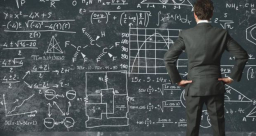Did you find an error or inaccuracy? Feel free to write us. Thank you!Tips to related online calculators
Do you have a linear equation or system of equations and looking for its solution? Or do you have a quadratic equation?

## Related math problems and questions:

• Equation 25Solve following simple equation: 3/4(x+5)=1/2(x+9)
• If x=4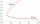If x=4 and y=-3, what is the value of the expression x² + 2xy - 4x + 3y + y²?
• FractionsSort fractions z1 = (6)/(11); z2 = (10)/(21); z3 = (19)/(22) by its size. Result write as three serial numbers 1,2,3.
• Equation 23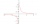Find value of unknown x in equation: x+3/x+1=5 (problem finding x)
• Evaluate expressionIf x=2, y=-5 and z=3 what is the value of x-2y
• Intersections 3Find the intersections of the circles x2 + y2 + 6 x - 10 y + 9 = 0 and x2 + y2 + 18 x + 4 y + 21 = 0
• Expressions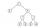Find out value of expressions if a = -1, b =2: x=b - 2a - ab y=a3 - b2 - 2ab z=a2 b3 - a3 b2 w=a + b + a3 - b2
• Cakes1/3 poppy cake, 1/3 apple, 15 pieces of cheese. How many are totally cakes?
• Expression plus minusEvaluate expression: (-1)2 . 12 – 6 : 3 + (-3) . (-2) + 22 – (-3) . 2
• EquationFind x: x + 1/2 = 1/3
• Denominator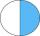Calculate the missing denominator x: (264)/(96) = (11)/(x)
• Expression 1What is 7+8-(5×2)+5-4+(6×(5-3)+6)-(8+10)-7+6?
• Eq2 equationsFor each of the following problems, determine the roots of the equation. Given the roots, sketch the graph and explain how your sketch matches the roots given and the form of the equation: g(x)=36x2-12x+5 h(x)=x2-4x+20 f(x)=4x2-24x+45 p(x)=9x2-36x+40
• Evaluate 5Evaluate expression x2−7x+12x−4 when x=−1
• Value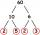Determine the value of the following exspressions: a) (23-25)·(4-5) b) (97-123):(18+8)
• Simple equation 1035= 7*3*x what is x?
• One kilogramThe apple weighs 125 grams and half apple. How many apples weigh 1 kilogram?